### Home > MC1 > Chapter 6 > Lesson 6.2.6 > Problem6-111

6-111.
1. . Simplify each expression below without using a calculator. Homework Help ✎1. 8 + (−6) + 4

2. −15 + 3 + 6

3. −8 + 12 − 2

4. 6(−8)

5. 5(−9)

6. −61 − (−13)

7. 4(−7)

8. 120 ÷ (−10)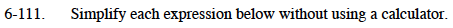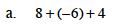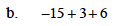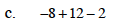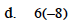Refer to problem 2-132 or a similar problem for help.

−6

2

6(8) = 48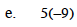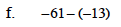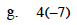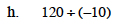−45

This is the same as −61 + 13. Can you explain why?

−28

−12